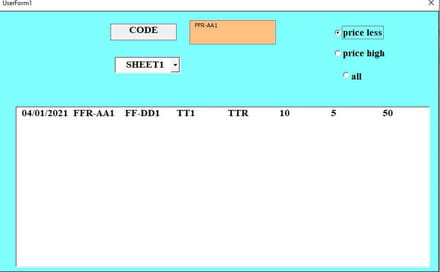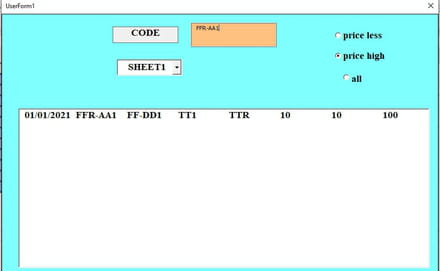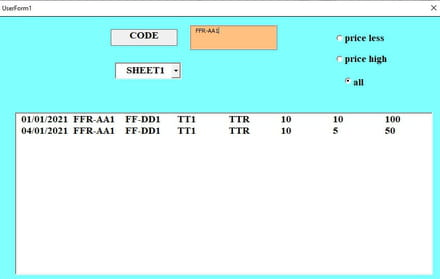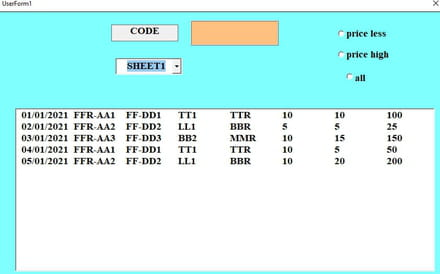# How can I link optionbutton and textbox based on selected from combobox

Solved/Closed
abdelfatah_0230 Posts 73 Registration date Thursday July 18, 2019 Status Member Last seen July 23, 2022 - Updated on Oct 25, 2021 at 06:59 AM
TrowaD Posts 2921 Registration date Sunday September 12, 2010 Status Moderator Last seen January 16, 2023 - Nov 4, 2021 at 11:47 AM
Hello,

hi
actually I got this codes from another web and mod some . it succeed and works , but my question how should link with the others tools ?
first I would select specific sheet form combobox and select price less and write the item in textbox based on COL B then should show all of data in listbox is relating of item is writing in textbox1 based on col B and brings the price less based on COL G(UNIT PRICE) .the same thing if I select price high should bring all of data which contain price less , and if I select all should show all of data for the item whether high or less with considering it shouldn't show any thing in listbox when run the userform .
it should show data in listbox after fill the combobox ,textbox, select option ,otherwise the listbox is empty .
note: if the item is no less or no high then should also show . I mean if the item contains 10 twice then should show
`Dim arrPrivate Sub UserForm_Initialize()With Sheets("sheet1")Set rg = .Range("A2:h15")arr = rgEnd With    With ListBox1    .ColumnCount = 8    .ColumnWidths = "80,120,80,80,80,80,80,80,80"    .List = arr    End WithEnd SubPrivate Sub textbox1_Change()Dim rw()    For i = 1 To UBound(arr)    If LCase(arr(i, 2)) Like "*" & LCase(TextBox1.Value) & "*" Then    ReDim Preserve rw(p)    rw(p) = Application.Index(arr, i, 0)    p = p + 1    End If    Next     If p = 0 Then MsgBox "NO MATCH": Exit SubWith ListBox1    If p > 1 Then        .List = Application.Transpose(Application.Transpose(rw))    Else        .Column = Application.Transpose(rw)    End IfEnd WithEnd Sub`

select sheet from combobox and write item in textbox1 based on column B and select price less based on column G

attach the cases to understand what I wantselect sheet from combobox and write item in textbox1 based on column B and select price less based on column Gselect sheet from combobox and write item in textbox1 based on column B and select price
high based on column Gselect sheet from combobox and write item in textbox1 based on column B and select all(whether prices high or less) based on column G
select sheet from combobox(show all of data which contain sheet )I know this is not easy to do that but I tried before issue this and I don't find solution in the internet
Related:

## 2 replies

TrowaD Posts 2921 Registration date Sunday September 12, 2010 Status Moderator Last seen January 16, 2023 547
Nov 2, 2021 at 12:42 PM
Hi Abdel,

I think I checked all the boxes with the code below. There is one thing I'm not sure what you want:
You select sheet1 and enter "code" ffr-aa1 and the respective data is show in the list.
Now you select sheet2 with "code" ffr-aa1 still in the textbox1.
1. Do you want to show all the data from sheet2 and clear the textbox1 data or
2. Do you want to show the data from sheet2 filtered with "code" ffr-aa1 and leave ffr-aa1 in textbox1.

I went with option 2, since you can just clear the textbox1 to see all data.

Do let me know if you want to go with option 1 or if I missed something else.

Here is the code:
```Dim arr

Private Sub ComboBox1_Change()
Dim tVal As String
If ComboBox1.Value = "SHEET1" Then
With Sheets("SHEET1")
Set rg = .Range("A2:H15")
arr = rg
End With
With ListBox1
.ColumnCount = 8
.ColumnWidths = "80;120;80;80;80;80;80;80"
.List = arr
End With
ElseIf ComboBox1.Value = "sheet2" Then
With Sheets("sheet2")
Set rg = .Range("A2:H15")
arr = rg
End With
With ListBox1
.ColumnCount = 8
.ColumnWidths = "80;120;80;80;80;80;80;80"
.List = arr
End With
End If
tVal = TextBox1.Value
TextBox1.Value = vbNullString
TextBox1.Value = tVal
End Sub

Private Sub OptionButton1_Click()
Dim i As Long
Dim minVal As Long
Dim rw()
minVal = Rows.Count

For i = 1 To UBound(arr)
If arr(i, 7) < minVal And LCase(arr(i, 2)) = LCase(TextBox1.Value) Then minVal = arr(i, 7)
Next i
For i = 1 To UBound(arr)
If arr(i, 7) = minVal And LCase(arr(i, 2)) = LCase(TextBox1.Value) Then
ReDim Preserve rw(p)
rw(p) = Application.Index(arr, i, 0)
p = p + 1
End If
Next i
With ListBox1
If p > 1 Then
.List = Application.Transpose(Application.Transpose(rw))
Else
.Column = Application.Transpose(rw)
End If
End With
End Sub

Private Sub OptionButton2_Click()
Dim i As Long
Dim maxVal As Long
Dim rw()

maxVal = 0

For i = 1 To UBound(arr)
If arr(i, 7) > maxVal And LCase(arr(i, 2)) = LCase(TextBox1.Value) Then maxVal = arr(i, 7)
Next i
For i = 1 To UBound(arr)
If arr(i, 7) = maxVal And LCase(arr(i, 2)) = LCase(TextBox1.Value) Then
ReDim Preserve rw(p)
rw(p) = Application.Index(arr, i, 0)
p = p + 1
End If
Next i
With ListBox1
If p > 1 Then
.List = Application.Transpose(Application.Transpose(rw))
Else
.Column = Application.Transpose(rw)
End If
End With
End Sub

Private Sub OptionButton3_Click()
Dim tVal As String
tVal = TextBox1.Value
TextBox1.Value = vbNullString
TextBox1.Value = tVal
End Sub

Private Sub textbox1_Change()
Dim rw()
For i = 1 To UBound(arr)
If LCase(arr(i, 2)) Like "*" & LCase(TextBox1.Value) & "*" Then
ReDim Preserve rw(p)
rw(p) = Application.Index(arr, i, 0)
p = p + 1
End If
Next

If p = 0 Then MsgBox "NO MATCH": Exit Sub
With ListBox1
If p > 1 Then
.List = Application.Transpose(Application.Transpose(rw))
Else
.Column = Application.Transpose(rw)
End If
End With
End Sub

Private Sub UserForm_Activate()
Dim WS As Worksheet
For Each WS In Worksheets
Next WS
End Sub```

Best regards,
Trowa
abdelfatah_0230 Posts 73 Registration date Thursday July 18, 2019 Status Member Last seen July 23, 2022
Updated on Nov 3, 2021 at 05:03 AM
Hi Trowa,
magnificant ! about this I went with option 2, since you can just clear the textbox1 to see all data. it's ok .
every things works as what I want .I no know how thank you . actually nobody answers me for my question for many tries in many forums . I know this is not easy to follow the code line by line to understand how should work and how should mod . you achieved a great work .
many thanks for your help .
have a nice day !
TrowaD Posts 2921 Registration date Sunday September 12, 2010 Status Moderator Last seen January 16, 2023 547 > abdelfatah_0230 Posts 73 Registration date Thursday July 18, 2019 Status Member Last seen July 23, 2022
Nov 4, 2021 at 11:47 AM
TrowaD Posts 2921 Registration date Sunday September 12, 2010 Status Moderator Last seen January 16, 2023 547
Oct 25, 2021 at 12:10 PM
Hi Abdel,

You write :
it should show data in listbox after fill the combobox ,textbox, select option ,otherwise the listbox is empty
select sheet from combobox(show all of data which contain sheet )
So which one is it?

With price high and price less you mean the max and min values, right?

What does the field reading CODE do?

How did you create the screenshots, when the results are not produced by code?

Best regards,
Trowa
abdelfatah_0230 Posts 73 Registration date Thursday July 18, 2019 Status Member Last seen July 23, 2022
Updated on Oct 25, 2021 at 02:42 PM
Hi Trowa

select sheet from combobox(show all of data which contain sheet )
So which one is it?

it depends on which select the sheet . I have two sheets (sheet1,sheet2) as in the code in my file . each sheet contain data . if I select specific sheet should show data is relating for selected sheet from combobox

With price high and price less you mean the max and min values, right?
yes
What does the field reading CODE do?

based on column B and column 2 in lisbox as show in sheet